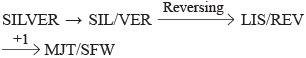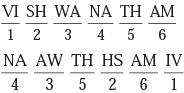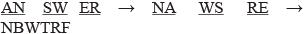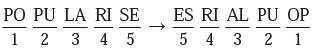Courses

# Olympiad Test: Coding – Decoding - 1

## 10 Questions MCQ Test Science Olympiad Class 6 | Olympiad Test: Coding – Decoding - 1

Description
This mock test of Olympiad Test: Coding – Decoding - 1 for Class 6 helps you for every Class 6 entrance exam. This contains 10 Multiple Choice Questions for Class 6 Olympiad Test: Coding – Decoding - 1 (mcq) to study with solutions a complete question bank. The solved questions answers in this Olympiad Test: Coding – Decoding - 1 quiz give you a good mix of easy questions and tough questions. Class 6 students definitely take this Olympiad Test: Coding – Decoding - 1 exercise for a better result in the exam. You can find other Olympiad Test: Coding – Decoding - 1 extra questions, long questions & short questions for Class 6 on EduRev as well by searching above.
QUESTION: 1

### If TRUTH is coded as SUQSTVSUGI, then the code for FALSE will be

Solution:

Each letter of the word ‘TRUTH’ is replaced by a set of two letters – one preceding it and the other following it – in the code. Thus, T is replaced by SU, R is replaced by QS and so on.

QUESTION: 2

### In a certain code, INACTIVE is written as VITCANIE. How is COMPUTER written in the same code?

Solution:

All the letters of the word, except the last letter, are written in a reverse order to obtain the code.

QUESTION: 3

### In a certain code, COVALENT, is written as BWPDUOFM and FORM is written as PGNS. How will SILVER be written in that code?

Solution:

Here,QUESTION: 4

In a certain code, VISHWANATHAN is written as NAAWTHHSANIV. How is KARUMAKARANA written in that code?

Solution:

Divide the given word into six sets of two letters each and label these sets from 1 to 6.
Then the code contains these sets in the order 4, 3, 5, 2, 6, 1 with the letters of sets 3, 2, 1 written in a reverse order. Thus, we have:QUESTION: 5

In a certain code, MOTHER is written as ONHURF. How will ANSWER be written in that code?

Solution:

Divide the word into three groups of two letters each and write the letters of each group in the reverse order.QUESTION: 6

In a certain code, the words COME AT ONCE, were written as XLNVZGLMXV. In the same code, which of the following would code OK?

Solution:

Each letter in the word is replaced by the letter which occupies the same position from the other end of the alphabet, to obtain the code.

QUESTION: 7

In a certain code, RAIL is written as KCTN and SPEAK is written as CGRUM. How will AVOID be written in that code?

Solution:

All the letters of the word, except the last letter, are written in the reverse order and in the group of letters so obtained each letter is moved into steps forward to get the code.

QUESTION: 8

If CONTRIBUTE is written as ETBUIRNTOC, then which letter will be in the sixth place when counted from the left it POPULARISE is written in the same way?

Solution:Clearly, the sixth letter from the left in the code is L.

QUESTION: 9

If in a certain code, GRASP is coded as BMVMK, which word would be coded as CRANE?

Solution:

Each letter of the word is five steps ahead the corresponding letter of the code.

QUESTION: 10

If NARGRUED is the code for GRANDEUR, which word is coded as SERPEVRE?

Solution:

The code has been obtained by writing the first four and the last four letters of the word in the reverse order. Thus, we have.
SERPEVRE → SERP/EVRE → PRES/ERVE → PRESERVE.